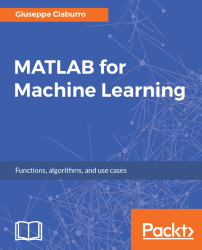•#### MATLAB for Machine Learning#### Overview of this book

MATLAB is the language of choice for many researchers and mathematics experts for machine learning. This book will help you build a foundation in machine learning using MATLAB for beginners. You’ll start by getting your system ready with t he MATLAB environment for machine learning and you’ll see how to easily interact with the Matlab workspace. We’ll then move on to data cleansing, mining and analyzing various data types in machine learning and you’ll see how to display data values on a plot. Next, you’ll get to know about the different types of regression techniques and how to apply them to your data using the MATLAB functions. You’ll understand the basic concepts of neural networks and perform data fitting, pattern recognition, and clustering analysis. Finally, you’ll explore feature selection and extraction techniques for dimensionality reduction for performance improvement. At the end of the book, you will learn to put it all together into real-world cases covering major machine learning algorithms and be comfortable in performing machine learning with MATLAB.
Title PageCreditsForewordwww.PacktPub.comCustomer FeedbackPrefaceFree Chapter
Getting Started with MATLAB Machine LearningImporting and Organizing Data in MATLABFrom Data to Knowledge DiscoveryFinding Relationships between Variables - Regression TechniquesPattern Recognition through Classification AlgorithmsIdentifying Groups of Data Using Clustering MethodsSimulation of Human Thinking - Artificial Neural NetworksImproving the Performance of the Machine Learning Model - Dimensionality ReductionMachine Learning in Practice## Chapter 4. Finding Relationships between Variables - Regression Techniques

Regression analysis is a statistical process of studying the relationship between a set of independent variables (explanatory variables) and the dependent variable (response variable). Through this technique, it is possible to understand how the value of the response variable changes when the explanatory variable is varied.

Consider a group of bikers about which some information has been collected: number of years of use, number of kilometers traveled in one year, and number of falls. Through these techniques, we can find that on an average, when the number of kilometers traveled increases, the number of falls also increases. By increasing the number of years of motorcycle usage and so increasing the experience, the number of falls tends to decrease.

A regression analysis can be conducted for a dual purpose:

• Explanatory, to understand and weigh the effects of the independent variable on the dependent variable according...